# Hill Cipher in Cryptography

Cryptography | Playfair Cipher: In this tutorial, we will briefly study the basic hill cipher and its examples aim to capture. We will cover the types of messages in hill cipher. By Monika Sharma Last updated : May 24, 2023

## What is Hill Cipher in Cryptography?

It is a polygraphic substitution cipher that depends on linear algebra. Every letter from the alphabet is represented by several modulo 26. Simply, we write as A = 0, B = 1, ..., Z = 25 is used, but this is not a correct feature of the cipher.

To encrypt a message, each block of n letters is multiplied by an invertible n × n matrix, against modulus 26 or we can say the multiple inverses of n.

To decrypt the message, every block is multiplied by the inverse of the matrix or inversed matrix used for encryption.

The matrix used for encryption is the plain text and one matrix is formed is cipher key when we combine or multiple them we get the new matrix called ciphertext, and the key should be chosen randomly from the set of invertible n × n matrices (modulo 26).

## Hill Cipher Encryption

We have to do encryption on the message 'ACT' (n=3). The key is 'GYBNQKURP' which can be written as the n x n matrix that is 3 x 3 matrix,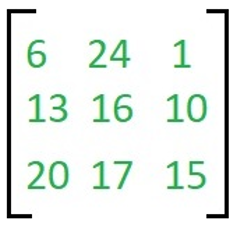The message for encryption 'ACT' is written as a vector,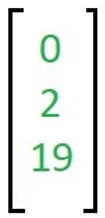The enciphered vector is given as,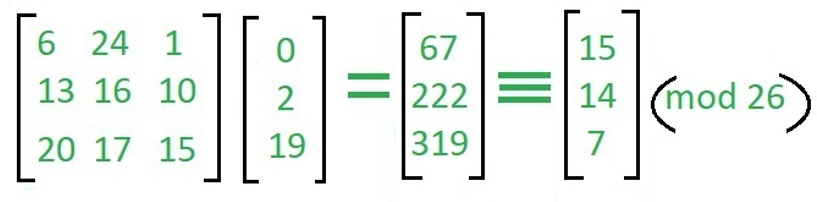In this, we just do the multiple matrices we just multiple the 1 row with 1 column as we can say 6*0+24*2+1*19=67 same as 13*0+16*2+10*19=222 and 20*0+17*2+15*19=319.

And that matrix does the mod 26 with particular column and made again the 3*1 matrix and we alphabetically write them with called ciphertext i.e "POH".

## Hill Cipher Decryption

To decrypt the message, we turn the ciphertext back into a plain text, then simply multiply by the inverse matrix of the key matrix as "IFKVIVVMI" in letters. The inverse of the matrix used in the encryption is,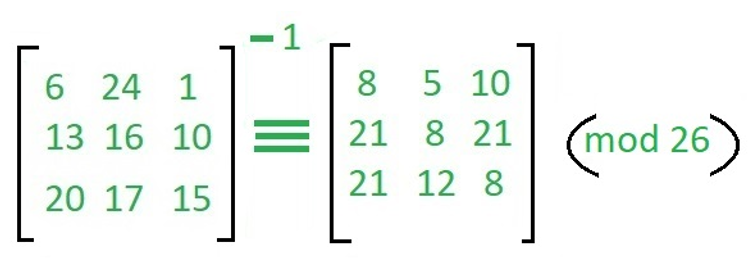For ciphertext which we already find we decrypt that and make plain text,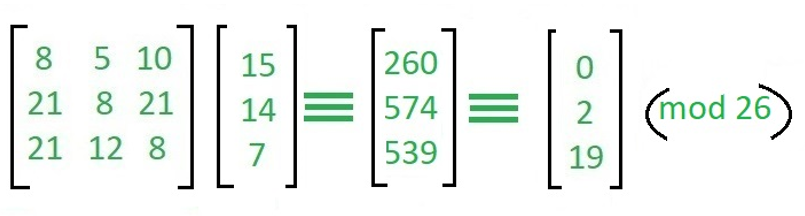Now, we just find the inverse matrix then multiple it by key then it will give us back "ACT".

Let, K is DDCF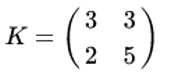be the key and suppose the plaintext is "HELP". Then this plaintext is represented by two pairs because n is 2.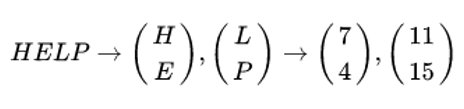Firstly we do encryption, then we will compute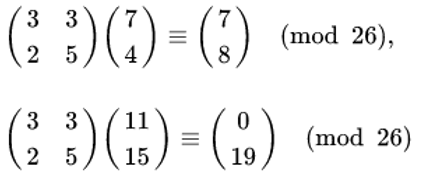and continue encryption as follows and written as,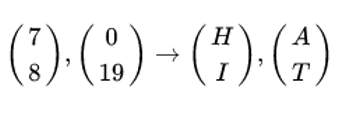The matrix K is invertible, hence K-1 exists such that KK-1 = K-1K = I2 The inverse of K can be computed by using the formula,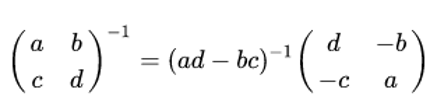This formula still holds after a modular reduction if a modular multiplicative inverse is used to compute.

Now, we have to do decryption,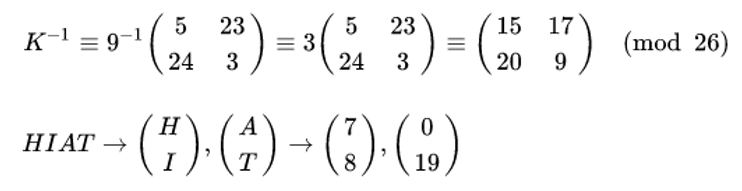Then we compute,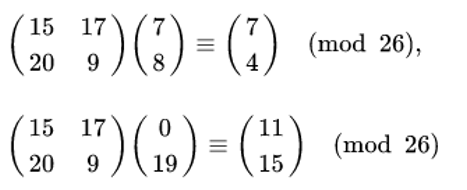And we get,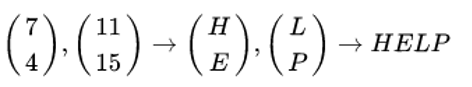## Hill Cipher Security

The basic Hill Cipher is vulnerable to a known-plaintext attack that attacks by key because it is completely linear algebra. An opposite site that intercepts n plaintext/ciphertext character pairs can set up a linear system that can be easily solved; if this will happen then this system is undefined, it is the only way is to add a few more plaintexts/ciphertext pairs. While when n x n matrix multiplication is a useful step when it will be combined with other non-linear operations because matrix multiplication can provide diffusion. For example, a unique chosen matrix can give security that minor differences before the matrix multiplication will give the answer in huge differences after the matrix multiplication. Otherwise, some new ciphers use a matrix multiplication step to gave diffusion. For example, the MixColumns matrix step in AES cipher is matrix multiplication. The function g in Twofish is a combination of non-linear algebra S-boxes i.e substitution boxes with a carefully chosen matrix multiplication (MDS) is used this.

Reference: Hill Cipher